(10 Kb)
Updated: 08-13-16 01:20 AM
Pictures
File Info
 Compatibility: Legion (7.0.3) Updated: 08-13-16 01:20 AM Created: 08-31-12 01:19 PM Downloads: 110,426 Favorites: 17 MD5:

# TBags with NewCurrencies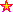Version: 7.01
by: 堂吉先生 [More]

this verson is based on DuffedUI's bag interface because no one fix cargbags
you can change currencies.lua as follows to make your wish of what to display

Bag.lua line 10 -13 set Bag size line 809 set bag position

for sell some armor and weapon ,add level type and short for the strings
location for your clients from line 16 to 59 and change line 641 to fit client

currencies.lua --货币显示currencis display
--可以使用下列数据自己排序1-N 推荐 N<=6 use the number to order 1-N suggest N<=6
Currency = {
 = 392, --Honor Points荣誉
 = 390, --Conquest Points征服
 = 994, --Seal of Tempered Fate钢化命运印记
 = 823, --Apexis Crystal埃匹希斯水晶
 = 824, --Garrison Resources要塞资源
 = 515, --Darkmoon Prize Ticket暗月票
 = 738, --Lesser Charm of Good Fortune
 = 944, --Artifact Fragment神器碎片
 = 810, --Black Iron Fragment黑铁碎片
 = 980, --Dingy Iron Coins肮脏的铁币
 = 789, --Bloody Coin染血的铸币
 = 241, --Champion's Seal冠军的印记
 = 402, --Ironpaw Token铁掌印记
 = 416, --Mark of the World Tree世界之树的印记
 = 777, --Timeless Coin永恒铸币
 = 391, --Tol Barad Commendation托尔巴拉德奖章
 = 776, --Warforged Seal战火铸币
}
local N = 7 --显示的货币数量 the amount of currencies to display

6.2
level fixed

6.06
armor and weapons 's level and type in zhCN or zhTW
6.04
armor and weapons 's level and type

6.03 bag movable
make BankItemsPerRow and BagItemsPerRow setable fix bankframe
fix bankframe bug with aurora
make 7 currencies for 6.0.2

5.2
.toc update
50102
fix font setting
50101
fix BUTTON setting problem
50100
update to 5.1
Optional Files (0)

Archived Files (1)
 File Name Version Size Author Date 7.0 10kB 堂吉先生 08-12-16 11:31 PM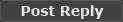Comment Options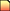08-16-16, 01:45 AM
A Cliff Giant

Forum posts: 74
Originally Posted by 堂吉先生
 Originally Posted by badness That is the new one that gave me the error. It happened when I tried to change my bags to add more bag space
local ButtonSetSize = 38
local ButtonSpacing = 1
local BagItemsPerRow = 12
local BankItemsPerRow = 18
what is BAG space? I DIDNT GET IT.
I replaced 20 slot bags with 30 slot bags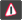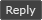08-16-16, 12:35 AM

 Originally Posted by badness That is the new one that gave me the error. It happened when I tried to change my bags to add more bag space
local ButtonSetSize = 38
local ButtonSpacing = 1
local BagItemsPerRow = 12
local BankItemsPerRow = 18
what is BAG space? I DIDNT GET IT.08-15-16, 10:30 AM badness A Cliff Giant Forum posts: 74 File comments: 54 Uploads: 0 That is the new one that gave me the error. It happened when I tried to change my bags to add more bag space08-15-16, 10:06 AM

 Originally Posted by badness The ButtonSpacing for the Banks/Reagents and Bags don't seem to hold the same values and I also got this error: Lua Code: `Message: Interface\AddOns\TBags\Bags.lua:676: attempt to index field 't' (a nil value)Time: 08/15/16 09:26:04Count: 1Stack: Interface\AddOns\TBags\Bags.lua:676: in function `SlotUpdate'Interface\AddOns\TBags\Bags.lua:726: in function `BagUpdate'Interface\AddOns\TBags\Bags.lua:926: in function  Locals: id = 4Button = ContainerFrame5Item23 { JunkIcon = { } NewItemTexture = { } BattlepayItemTexture = { } 0 =  UpdateTooltip = defined @Interface\FrameXML\ContainerFrame.lua:1080 IsSkinned = true count = 0 flash = { } newitemglowAnim = { } Count = ContainerFrame5Item23Count { } IconBorder = { } flashAnim = { } searchOverlay = ContainerFrame5Item23SearchOverlay { } icon = ContainerFrame5Item23IconTexture { } SplitStack = defined @Interface\FrameXML\ContainerFrame.lua:909}ItemLink = nil_ = nil_ = nilLock = nilIsQuestItem = nil(*temporary) = nil(*temporary) = nil(*temporary) = "8"(*temporary) = ContainerFrame5Item23 { JunkIcon = { } NewItemTexture = { } BattlepayItemTexture = { } 0 =  UpdateTooltip = defined @Interface\FrameXML\ContainerFrame.lua:1080 IsSkinned = true count = 0 flash = { } newitemglowAnim = { } Count = ContainerFrame5Item23Count { } IconBorder = { } flashAnim = { } searchOverlay = ContainerFrame5Item23SearchOverlay { } icon = ContainerFrame5Item23IconTexture { } SplitStack = defined @Interface\FrameXML\ContainerFrame.lua:909}(*temporary) = 8(*temporary) = nil(*temporary) = nil(*temporary) = nil(*temporary) = nil(*temporary) = nil(*temporary) = nil(*temporary) = nil(*temporary) = nil(*temporary) = nil(*temporary) = nil(*temporary) = "attempt to index field 't' (a nil value)"Texts = { 53 = 1 43 = 1 73 = 1 63 = 1 93 = 1 83 = 1 13 = 1 3 = 1 33 = 1 23 = 1 42 = 1 52 = 1 62 = 1 72 = 1 82 = 1 92 = 1 329 = 1 1114 = 1 429 = 1 2 = 1 12 = 1 22 = 1 32 = 1 -115 = 1 1115 = 1 1128 = 1 -128 = 1 -125 = 1 1125 = 1 328 = 1 1127 = 1 -127 = 1 87 = 1 77 = 1 1018 = 1 47 = 1 37 = 1 67 = 1 57 = 1 1028 = 1 -15 = 1 15 = 1 115 = 1 215 = 1 315 = 1 415 = 1 515 = 1 615 = 1 715 = 1 7 = 1 14 = 1 314 = 1 17 = 1 514 = 1 414 = 1 714 = 1 614 = 1 107 = 1 86 = 1 96 = 1 36 = 1 46 = 1 56 = 1 66 = 1 106 = 1 -116 = 1 1117 = 1 1116 = 1 -117 = 1 6 = 1 16 = 1 26 = 1 424 = 1 -17 = 1 71 = 1 61 = 1 1010 = 1 41 = 1 -113 = 1 91 = 1 81 = 1 1113 = 1 1020 = 1 -120 = 1 1124 = 1 31 = 1 21 = 1 11 = 1 1 = 1 914 = 1 814 = 1 1110 = 1 1120 = 1 -110 = 1 105 = 1 1024 = 1 -126 = 1 1030 = 1 1126 = 1 -123 = 1 -121 = 1 1012 = 1 1121 = 1 -119 = 1 -111 = 1 1022 = 1 1111 = 1 1119 = 1 1026 = 1 330 = 1 430 = 1 1129 = 1 -112 = 1 1112 = 1 1021 = 1 -122 = 1 422 = 1 322 = 1 222 = 1 122 = 1 1011 = 1 321 = 1 421 = 1 121 = 1 221 = 1 1118 = 1 217 = 1 1025 = 1 85 = 1 75 = 1 820 = 1 720 = 1 213 = 1 920 = 1 316 = 1 216 = 1 817 = 1 917 = 1 716 = 1 713 = 1 516 = 1 416 = 1 118 = 1 218 = 1 916 = 1 816 = 1 -18 = 1 1130 = 1 15 = 1 5 = 1 410 = 1 110 = 1 210 = 1 10 = 1 94 = 1 74 = 1 84 = 1 54 = 1 64 = 1 34 = 1 1023 = 1 326 = 1 426 = 1 102 = 1 1029 = 1 101 = 1 420 = 1 24 = 1 1019 = 1 4 = 1 220 = 1 120 = 1 -12 = 1 312 = 1 412 = 1 512 = 1 612 = 1 12 = 1 112 = 1 212 = 1 611 = 1 919 = 1 411 = 1 311 = 1 211 = 1 812 = 1 11 = 1 719 = 1 219 = 1 119 = 1 419 = 1 319 = 1 1017 = 1 911 = 1 811 = 1 711 = 1 323 = 1 324 = 1 325 = 1 910 = 1 327 = 1 103 = 1 810 = 1 918 = 1 423 = 1 1015 = 1 425 = 1 427 = 1 428 = 1 718 = 1 818 = 1 -124 = 1 108 = 1 511 = 1 -114 = 1 -19 = 1 -16 = 1 -14 = 1 510 = 1 717 = 1 -11 = 1 51 = 1 -13 = 1 613 = 1 55 = 1 214 = 1 27 = 1 313 = 1 -118 = 1 513 = 1`08-14-16, 04:04 PM badness A Cliff Giant Forum posts: 74 File comments: 54 Uploads: 0 The ButtonSpacing for the Banks/Reagents and Bags don't seem to hold the same values and I also got this error: Lua Code: `Message: Interface\AddOns\TBags\Bags.lua:676: attempt to index field 't' (a nil value)Time: 08/15/16 09:26:04Count: 1Stack: Interface\AddOns\TBags\Bags.lua:676: in function `SlotUpdate'Interface\AddOns\TBags\Bags.lua:726: in function `BagUpdate'Interface\AddOns\TBags\Bags.lua:926: in function  Locals: id = 4Button = ContainerFrame5Item23 { JunkIcon = { } NewItemTexture = { } BattlepayItemTexture = { } 0 =  UpdateTooltip = defined @Interface\FrameXML\ContainerFrame.lua:1080 IsSkinned = true count = 0 flash = { } newitemglowAnim = { } Count = ContainerFrame5Item23Count { } IconBorder = { } flashAnim = { } searchOverlay = ContainerFrame5Item23SearchOverlay { } icon = ContainerFrame5Item23IconTexture { } SplitStack = defined @Interface\FrameXML\ContainerFrame.lua:909}ItemLink = nil_ = nil_ = nilLock = nilIsQuestItem = nil(*temporary) = nil(*temporary) = nil(*temporary) = "8"(*temporary) = ContainerFrame5Item23 { JunkIcon = { } NewItemTexture = { } BattlepayItemTexture = { } 0 =  UpdateTooltip = defined @Interface\FrameXML\ContainerFrame.lua:1080 IsSkinned = true count = 0 flash = { } newitemglowAnim = { } Count = ContainerFrame5Item23Count { } IconBorder = { } flashAnim = { } searchOverlay = ContainerFrame5Item23SearchOverlay { } icon = ContainerFrame5Item23IconTexture { } SplitStack = defined @Interface\FrameXML\ContainerFrame.lua:909}(*temporary) = 8(*temporary) = nil(*temporary) = nil(*temporary) = nil(*temporary) = nil(*temporary) = nil(*temporary) = nil(*temporary) = nil(*temporary) = nil(*temporary) = nil(*temporary) = nil(*temporary) = "attempt to index field 't' (a nil value)"Texts = { 53 = 1 43 = 1 73 = 1 63 = 1 93 = 1 83 = 1 13 = 1 3 = 1 33 = 1 23 = 1 42 = 1 52 = 1 62 = 1 72 = 1 82 = 1 92 = 1 329 = 1 1114 = 1 429 = 1 2 = 1 12 = 1 22 = 1 32 = 1 -115 = 1 1115 = 1 1128 = 1 -128 = 1 -125 = 1 1125 = 1 328 = 1 1127 = 1 -127 = 1 87 = 1 77 = 1 1018 = 1 47 = 1 37 = 1 67 = 1 57 = 1 1028 = 1 -15 = 1 15 = 1 115 = 1 215 = 1 315 = 1 415 = 1 515 = 1 615 = 1 715 = 1 7 = 1 14 = 1 314 = 1 17 = 1 514 = 1 414 = 1 714 = 1 614 = 1 107 = 1 86 = 1 96 = 1 36 = 1 46 = 1 56 = 1 66 = 1 106 = 1 -116 = 1 1117 = 1 1116 = 1 -117 = 1 6 = 1 16 = 1 26 = 1 424 = 1 -17 = 1 71 = 1 61 = 1 1010 = 1 41 = 1 -113 = 1 91 = 1 81 = 1 1113 = 1 1020 = 1 -120 = 1 1124 = 1 31 = 1 21 = 1 11 = 1 1 = 1 914 = 1 814 = 1 1110 = 1 1120 = 1 -110 = 1 105 = 1 1024 = 1 -126 = 1 1030 = 1 1126 = 1 -123 = 1 -121 = 1 1012 = 1 1121 = 1 -119 = 1 -111 = 1 1022 = 1 1111 = 1 1119 = 1 1026 = 1 330 = 1 430 = 1 1129 = 1 -112 = 1 1112 = 1 1021 = 1 -122 = 1 422 = 1 322 = 1 222 = 1 122 = 1 1011 = 1 321 = 1 421 = 1 121 = 1 221 = 1 1118 = 1 217 = 1 1025 = 1 85 = 1 75 = 1 820 = 1 720 = 1 213 = 1 920 = 1 316 = 1 216 = 1 817 = 1 917 = 1 716 = 1 713 = 1 516 = 1 416 = 1 118 = 1 218 = 1 916 = 1 816 = 1 -18 = 1 1130 = 1 15 = 1 5 = 1 410 = 1 110 = 1 210 = 1 10 = 1 94 = 1 74 = 1 84 = 1 54 = 1 64 = 1 34 = 1 1023 = 1 326 = 1 426 = 1 102 = 1 1029 = 1 101 = 1 420 = 1 24 = 1 1019 = 1 4 = 1 220 = 1 120 = 1 -12 = 1 312 = 1 412 = 1 512 = 1 612 = 1 12 = 1 112 = 1 212 = 1 611 = 1 919 = 1 411 = 1 311 = 1 211 = 1 812 = 1 11 = 1 719 = 1 219 = 1 119 = 1 419 = 1 319 = 1 1017 = 1 911 = 1 811 = 1 711 = 1 323 = 1 324 = 1 325 = 1 910 = 1 327 = 1 103 = 1 810 = 1 918 = 1 423 = 1 1015 = 1 425 = 1 427 = 1 428 = 1 718 = 1 818 = 1 -124 = 1 108 = 1 511 = 1 -114 = 1 -19 = 1 -16 = 1 -14 = 1 510 = 1 717 = 1 -11 = 1 51 = 1 -13 = 1 613 = 1 55 = 1 214 = 1 27 = 1 313 = 1 -118 = 1 513 = 1` Last edited by badness : 08-15-16 at 07:28 AM.08-14-16, 10:07 AM Tweeker A Cobalt MageweaverForum posts: 234 File comments: 153 Uploads: 5 English version? Sorry cant speak asian __________________08-05-16, 05:30 PM badness A Cliff Giant Forum posts: 74 File comments: 54 Uploads: 0 any chance of an update for legion?07-27-15, 01:11 PM agneswww A Kobold Labourer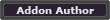Forum posts: 0 File comments: 21 Uploads: 1 zhTW elseif (GetLocale() == "zhTW") then L = {}; WEAPON = "武器" ARMOR = "護甲" L["布甲"]="布" L["皮甲"]="皮" L["锁甲"]="鎖" L["板甲"]="鎧" L["INVTYPE_SHIELD"]="盾" L["INVTYPE_HOLDABLE"]="副手" L["INVTYPE_HEAD"]="頭" L["INVTYPE_SHOULDER"]="肩" L["INVTYPE_CHEST"]="胸" L["INVTYPE_WAIST"]="腰" L["INVTYPE_LEGS"]="腿" L["INVTYPE_FEET"]="腳" L["INVTYPE_WRIST"]="腕" L["INVTYPE_HAND"]="手" L["INVTYPE_WEAPONOFFHAND"]="副武"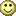12-08-12, 01:00 PM EridaNuuS A Kobold Labourer Forum posts: 1 File comments: 44 Uploads: 0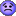return to bagnon ((( Code: ```Message: Interface\AddOns\TBags\Bags.lua:251: attempt to call global 'UpdateJusticeInfo' (a nil value) Time: Sat Dec 8 20:57:44 2012 Count: 1 Stack: Interface\AddOns\TBags\Bags.lua:251: in function `CurrInfos' Interface\AddOns\TBags\Bags.lua:247: in function [C]: in function `LoadAddOn' [string "Interface\FrameXML\BasicControls.xml: [C]: ? [C]: in function `SetFont' Interface\AddOns\TBags\Bags.lua:264: in function `OnCreate' Interface\AddOns\TBags\cargBags\base\container.lua:56: in function `New' Interface\AddOns\TBags\Bags.lua:47: in function `OnInit' ...erface\AddOns\TBags\cargBags\base\implementation.lua:251: in function `Init' ...erface\AddOns\TBags\cargBags\base\implementation.lua:75: in function `?' Interface\AddOns\TBags\cargBags\base\core.lua:173: in function [C]: in function `Show' ...erface\AddOns\TBags\cargBags\base\implementation.lua:104: in function `Toggle' Interface\AddOns\TBags\cargBags\base\core.lua:74: in function `ToggleBackpack' Interface\AddOns\TBags\cargBags\base\core.lua:145: in function Locals: (*temporary) = nil (*temporary) = "attempt to call global 'UpdateJusticeInfo' (a nil value)"```12-08-12, 05:07 AM

Re: Re: new error :(

Originally Posted by EridaNuuS
 Originally Posted by EridaNuuS I have new error Code: ```Message: Interface\AddOns\TBags\Bags.lua:249: attempt to call global 'UpdateJusticeInfo' (a nil value) Time: Sat Dec 8 02:43:13 2012 Count: 1 Stack: Interface\AddOns\TBags\Bags.lua:249: in function `CurrInfos' Interface\AddOns\TBags\Bags.lua:245: in function [C]: in function `LoadAddOn' [string "Interface\FrameXML\BasicControls.xml: [C]: ? [C]: in function `SetFont' Interface\AddOns\TBags\Bags.lua:262: in function `OnCreate' Interface\AddOns\TBags\cargBags\base\container.lua:56: in function `New' Interface\AddOns\TBags\Bags.lua:45: in function `OnInit' ...erface\AddOns\TBags\cargBags\base\implementation.lua:251: in function `Init' ...erface\AddOns\TBags\cargBags\base\implementation.lua:75: in function `?' Interface\AddOns\TBags\cargBags\base\core.lua:173: in function [C]: in function `Show' ...erface\AddOns\TBags\cargBags\base\implementation.lua:104: in function `Toggle' Interface\AddOns\TBags\cargBags\base\core.lua:74: in function `ToggleBackpack' Interface\AddOns\TBags\cargBags\base\core.lua:145: in function Locals: (*temporary) = nil (*temporary) = "attempt to call global 'UpdateJusticeInfo' (a nil value)"```

I 90 lvl and old version work fine.
you mean 50100 is oK while 50101 has BUG?12-07-12, 07:10 PM
EridaNuuS
A Kobold Labourer

Forum posts: 1
Re: new error :(

 Originally Posted by EridaNuuS I have new error Code: ```Message: Interface\AddOns\TBags\Bags.lua:249: attempt to call global 'UpdateJusticeInfo' (a nil value) Time: Sat Dec 8 02:43:13 2012 Count: 1 Stack: Interface\AddOns\TBags\Bags.lua:249: in function `CurrInfos' Interface\AddOns\TBags\Bags.lua:245: in function [C]: in function `LoadAddOn' [string "Interface\FrameXML\BasicControls.xml: [C]: ? [C]: in function `SetFont' Interface\AddOns\TBags\Bags.lua:262: in function `OnCreate' Interface\AddOns\TBags\cargBags\base\container.lua:56: in function `New' Interface\AddOns\TBags\Bags.lua:45: in function `OnInit' ...erface\AddOns\TBags\cargBags\base\implementation.lua:251: in function `Init' ...erface\AddOns\TBags\cargBags\base\implementation.lua:75: in function `?' Interface\AddOns\TBags\cargBags\base\core.lua:173: in function [C]: in function `Show' ...erface\AddOns\TBags\cargBags\base\implementation.lua:104: in function `Toggle' Interface\AddOns\TBags\cargBags\base\core.lua:74: in function `ToggleBackpack' Interface\AddOns\TBags\cargBags\base\core.lua:145: in function Locals: (*temporary) = nil (*temporary) = "attempt to call global 'UpdateJusticeInfo' (a nil value)"```

I 90 lvl and old version work fine.12-07-12, 06:45 PM EridaNuuS A Kobold Labourer Forum posts: 1 File comments: 44 Uploads: 0new error :( I have new error Code: ```Message: Interface\AddOns\TBags\Bags.lua:249: attempt to call global 'UpdateJusticeInfo' (a nil value) Time: Sat Dec 8 02:43:13 2012 Count: 1 Stack: Interface\AddOns\TBags\Bags.lua:249: in function `CurrInfos' Interface\AddOns\TBags\Bags.lua:245: in function [C]: in function `LoadAddOn' [string "Interface\FrameXML\BasicControls.xml: [C]: ? [C]: in function `SetFont' Interface\AddOns\TBags\Bags.lua:262: in function `OnCreate' Interface\AddOns\TBags\cargBags\base\container.lua:56: in function `New' Interface\AddOns\TBags\Bags.lua:45: in function `OnInit' ...erface\AddOns\TBags\cargBags\base\implementation.lua:251: in function `Init' ...erface\AddOns\TBags\cargBags\base\implementation.lua:75: in function `?' Interface\AddOns\TBags\cargBags\base\core.lua:173: in function [C]: in function `Show' ...erface\AddOns\TBags\cargBags\base\implementation.lua:104: in function `Toggle' Interface\AddOns\TBags\cargBags\base\core.lua:74: in function `ToggleBackpack' Interface\AddOns\TBags\cargBags\base\core.lua:145: in function Locals: (*temporary) = nil (*temporary) = "attempt to call global 'UpdateJusticeInfo' (a nil value)"```11-23-12, 02:59 AM

 Originally Posted by EridaNuuS Do not work new update (((( Code: `Message: Interface\AddOns\TBags\Bags.lua:249: attempt to call global 'UpdateJusticeInfo' (a nil value)`
this problem maybe come out when you are blow about 15 level dispear when you are over 15 level
fixed
Last edited by 堂吉先生 : 11-23-12 at 03:46 AM.11-21-12, 03:44 PM EridaNuuS A Kobold Labourer Forum posts: 1 File comments: 44 Uploads: 0 Do not work new update (((( Code: ```Message: Interface\AddOns\TBags\Bags.lua:249: attempt to call global 'UpdateJusticeInfo' (a nil value) Time: Wed Nov 21 23:42:57 2012 Count: 1 Stack: Interface\AddOns\TBags\Bags.lua:249: in function `CurrInfos' Interface\AddOns\TBags\Bags.lua:245: in function [C]: in function `LoadAddOn' [string "Interface\FrameXML\BasicControls.xml: [C]: ? [C]: in function `SetFont' Interface\AddOns\TBags\Bags.lua:262: in function `OnCreate' Interface\AddOns\TBags\cargBags\base\container.lua:56: in function `New' Interface\AddOns\TBags\Bags.lua:45: in function `OnInit' ...erface\AddOns\TBags\cargBags\base\implementation.lua:251: in function `Init' ...erface\AddOns\TBags\cargBags\base\implementation.lua:75: in function `?' Interface\AddOns\TBags\cargBags\base\core.lua:174: in function [C]: in function `Show' ...erface\AddOns\TBags\cargBags\base\implementation.lua:104: in function `Toggle' Interface\AddOns\TBags\cargBags\base\core.lua:74: in function `ToggleBag' Interface\FrameXML\ContainerFrame.lua:95: in function `ToggleBackpack' Interface\AddOns\TBags\cargBags\base\core.lua:146: in function Locals: (*temporary) = nil (*temporary) = "attempt to call global 'UpdateJusticeInfo' (a nil value)"```09-24-12, 06:08 AM

 Originally Posted by Panzervi what is the config command?
sorry no config ,change bags.lua,line 46 for bag columns ,line 56 for bank columns
alt+click to moveReport this File

Category Jump: